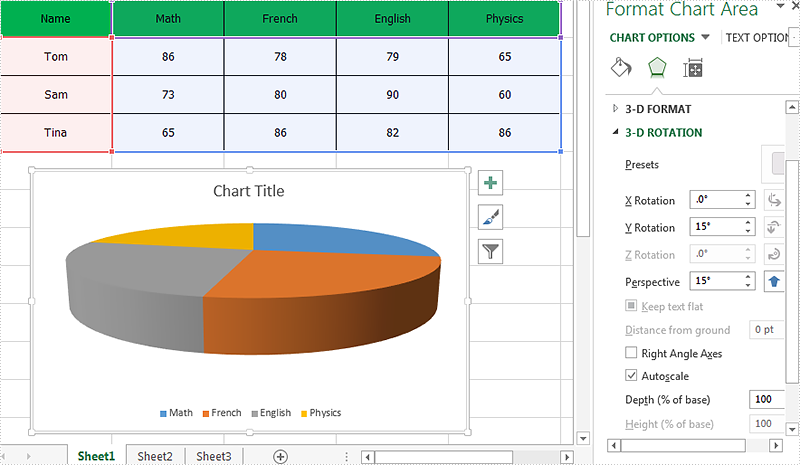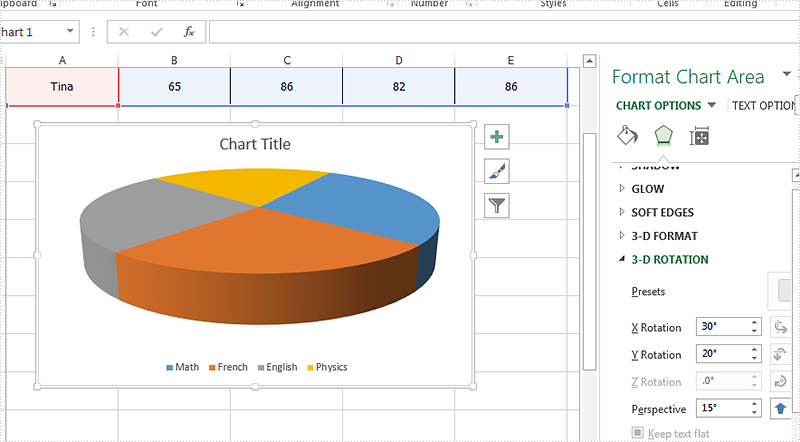How to set the rotation for the 3D chart on Excel in C#

With Spire.XLS, we can set the 3-D rotation easily in C#. The following code example explains how to set the rotation of the 3D chart view in C#. We will use 3D pie chart for example. Firstly, view the original 3D pie chart:Code snippet of how to set the 3D rotation for Excel Chart:

Step 1: Create a new instance of workbook and load the sample document from file.

Workbook workbook = new Workbook();

Step 2: Get the chart from the first worksheet.

Worksheet sheet = workbook.Worksheets;
Chart chart = sheet.Charts;

Step 3: Set Rotation of the 3D chart view for X and Y.

//X rotation:
chart.Rotation=30;
//Y rotation:
chart.Elevation = 20;

Step 4: Save the document to file.

workbook.SaveToFile("Result.xlsx", ExcelVersion.Version2010);

Effective screenshot of the Excel 3D chart rotation:Full codes:

using Spire.Xls;
namespace SetRotation
{

class Program
{

static void Main(string[] args)
{
Workbook workbook = new Workbook();

Worksheet sheet = workbook.Worksheets;
Chart chart = sheet.Charts;

//X rotation:
chart.Rotation = 30;
//Y rotation:
chart.Elevation = 20;

workbook.SaveToFile("Result.xlsx", ExcelVersion.Version2010);
}
}
}